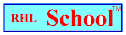Name_______________________________________________Date_________________________
Mathematics Problem Solving
Volume 4, Number 20, February 8, 1999
www.rhlschool.com

Perimeters and Shapes

Use a dictionary or the glossary of your math textbook to help you with any words that are new to you.

1. What is the perimeter of a regular hexagon that is four inches long on each side?

2. If a square had the same perimeter as the above hexagon, how long would each side be?

3. How long is each side of a regular pentagon if the pentagon’s perimeter is 60 meters?

4. Ryan made a rectangle out of construction paper. The perimeter of the rectangle was 20 centimeters. Ryan measured one of the sides and found it to be 7 centimeters long.

Cheryl made a rectangle out of construction paper. It also had a perimeter of 20 centimeters.

Only one of the rectangles was a square. Was it Ryan’s or Cheryl’s?

5. Michael made a regular octagon out of cardboard. It had a perimeter of 56 inches. Anne also made a regular octagon. Each side of her octagon was 7 inches long. Which one of the following must be true?

a. Both figures were made out of cardboard.
b. Both figures were perpendicular.
c. Both figures were asymmetric.
d. Both figures were congruent.
e. None of the above.